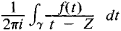# Cauchy Integral

## Cauchy Integral

an integral of the formwhere γ is a simple closed rectifiable curve in a complex plane and f(t) is a function of the complex variable t analytic on γ and in the interior of γ. If the point z lies within γ, then the Cauchy integral is equal to f(z). Thus, the Cauchy integral expresses the values of the analytic function f inside γ in terms of the values of f on γ. The integral was first examined by A. Cauchy in 1831.

Cauchy-type integrals are generalizations of the Cauchy integral. They have the same form, but the curve γ is not assumed to be closed and the function f(t) is not assumed to be analytic. Such integrals also define analytic functions. Their values on γ generally differ from the function f(t).

The systematic study of these integrals was begun by I. V. Sokhotskii and was subsequently continued chiefly by Russian and Soviet mathematicians (Iu. G. Kolosov, V. V. Golubev, I. I. Privalov, and N. I. Muskhelishvili). These studies led to further generalizations as well as to applications to problems of mechanics.

### REFERENCES

Markushevich, A. I. Teoriia analiticheskikh funktsii, 2nd ed., vols. 1–2. Moscow, 1967–68.
Privalov, I.I. Granichnye svoistva analiticheskikh funktsii, 2nd ed. Moscow-Leningrad, 1950.
References in periodicals archive ?
Let the approximate solution at the points of reference curves calculated by the Cauchy integral method be [u.sub.[epsilon]j](t), j = 0, 1.
Krishan, "Difference of composition operators from the space of Cauchy integral transforms to the Dirichlet space," Complex Analysis and Operator Theory, vol.
Then equality (3.1), the Cauchy integral formula and (3.4) show that
Sommen, "Cauchy Integral Formulae in Quaternionic Hermitean Clifford Analysis," Complex Analysis and Operator Theory, vol.
Shiffman's proof was based on Besicovitch's result, Theorem 1 above, on coordinate rotation, on the use of Cauchy integral formula and on the following result of Federer:
Cauchy integral representation for a region [OMEGA] is given as:
Substituting relation (12) to the first formulas (10) and (11) and using the Cauchy integral theorem, we get general formulas for the desired functions:
Of most importance to this work is the Cauchy integral. First, for a function f : t [right arrow] R, we say F is a [DELTA]-antiderivative of f on t, if [F.sup.[DELTA]](t) = f(t) for all t [member of] [T.sup.k].
The Cauchy integral formula for analytic functions cannot thus be used, and hence the CVBEM method given in (Hromadka II., T.V., 1984) cannot be either applied.
Emphasizing how complex analysis is a natural outgrowth of multivariable real calculus, this graduate textbook introduces the Cauchy integral formula, the properties and behavior of holomorphic functions, harmonic functions, analytic continuation, topology, Mergelyan's theorem, Hilbert spaces, and the prime number theorem.
We first study some properties of a regular function and then generalize Cauchy integral theorem and Cauchy integral formula in [A.sub.n](R).
Writing for undergraduate students of complex analysis, Bell updates his account of recent developments regarding the Cauchy integral formula, especially how new formulas expressing the classical objects of potential theory and conformal mapping in terms of Cauchy transforms and Szeg&lt;"o&gt; projections have led to new numerical methods for computing these objects.

Site: Follow: Share:
Open / Close